﻿ A Novel Fast FEA No-Load Loss Calculation Method for Stacked Core Three Phase Distribution Transformers

### A Novel Fast FEA No-Load Loss Calculation Method for Stacked Core Three Phase Distribution Transform...

Ehsan Hajipour, Mehdi Vakilian, Mohsen GhafouriOPEN ACCESSPEER-REVIEWED

## A Novel Fast FEA No-Load Loss Calculation Method for Stacked Core Three Phase Distribution Transformers

Ehsan Hajipour1,, Mehdi Vakilian1, Mohsen Ghafouri1

1Electrical Engineering Department, Sharif University of Technology, Tehran, Iran

### Abstract

Almost one third of the overall transmission and distribution systems energy losses are lost in distribution transformers, while its core losses account for almost 70% of the total transformers losses. A reasonably fast calculation tool to facilitate the core loss optimization through accurate predetermination of the impact of different design parameters on its losses seems indispensable, for a transformer manufacturer. In this paper, a novel fast 2-D FEA transformer core loss determination method is presented. Assuming the normal component of the magnetic field to be zero, this method reduces the computation time substantially in comparison with the available conventional methods (maintaining the same accuracy level). Employing Gaussian fitting curve, the anisotropic and the non-linear behavior of the magnetic core material are modeled. The extra core losses which occur in the core sheets joints and the stacking holes are simulated accurately in this work. The proposed method is implemented on 12 different commercial size distribution transformers, to verify the speed and the accuracy of this computation method.

### At a glance: Figures

123
Prev Next

• Hajipour, Ehsan, Mehdi Vakilian, and Mohsen Ghafouri. "A Novel Fast FEA No-Load Loss Calculation Method for Stacked Core Three Phase Distribution Transformers." International Transaction of Electrical and Computer Engineers System 2.1 (2014): 34-38.
• Hajipour, E. , Vakilian, M. , & Ghafouri, M. (2014). A Novel Fast FEA No-Load Loss Calculation Method for Stacked Core Three Phase Distribution Transformers. International Transaction of Electrical and Computer Engineers System, 2(1), 34-38.
• Hajipour, Ehsan, Mehdi Vakilian, and Mohsen Ghafouri. "A Novel Fast FEA No-Load Loss Calculation Method for Stacked Core Three Phase Distribution Transformers." International Transaction of Electrical and Computer Engineers System 2, no. 1 (2014): 34-38.

 Import into BibTeX Import into EndNote Import into RefMan Import into RefWorks

### 1. Introduction

Distribution transformers absorb the largest share in the power system distribution capital investments while they are also the most important elements of this section . These transformers with power efficiency often greater than 98% have the highest efficiency and reliability among the various power network devices . However, a significant portion of losses of transmission and distribution network is the continuous losses of transformers which exist even in the no-load state . Analyses of 25 European countries (EU-25) indicate that these losses in distribution transformers are almost one-third of the total transmission and distribution losses . In recent years, due to the more serious environmental concerns and the fast rise in the energy costs, the demands for efficient transformers have been increased considerably . In EU-25, core loss accounts for almost 70% of transformer total losses while the operating (or energy) efficiency is 93.38% . Thus a world-wide concern on no-load losses reduction is justified . To reduce these losses, different methods are introduced in the literatures [4, 9]. Apart from using better core materials, an improved core design can reduce these losses greatly . Thus it is inevitable for a transformer manufacturer to employ an accurate calculation tool to determine the impact of the design parameters on the core losses . This computational tool should model the core characteristics accurately and satisfy other requirements as follows [8, 10]:

Ÿ  Anisotropy and non-linearity of the magnetic core material;

Ÿ  A voltage boundary condition similar to the standard no-load test ;

Ÿ  Core losses in directions other than the rolling direction of the core material;

Ÿ  Joints and the extra core losses resulted from the air gaps which caused the distortion of flux distribution [13, 14];

Ÿ  Stacking holes.

Since the magnetic field distribution in transformer core and surrounding space has three-dimensional (3-D) nature, the exact modeling of transformer core for studying the impact of various design parameters, requires a 3-D model . Solving 3-D models provides several problems such as very long simulation time and needs great amounts of computer resources (RAM, hard disk) . Especially exact 3-D modeling of the core with its laminations becomes impossible. Considering these problems, engineers and transformer designers try to replace the 3-D core model with some two-dimensional (2-D) simulations [8, 9, 10, 12]. Applying 2-D models decreases the computation time and complexity of problem significantly . In 2-D modeling of core loss, trade-off between the accuracy and the computation time results in various 2-D models. Which need high computation time and often determine core loss accurately, however the transformer core optimization software have less opportunity to evaluate the various modes of design parameters. Thus, the resulted design may not be the most efficient one.

In this paper, a new 2-D FEA transformer core loss estimation method is introduced. This method, while maintains the accuracy at an acceptable level, reduces the computation time substantially when compared with the conventional methods. For example in , although its estimation accuracy is high however, every single pocket of the core must be modeled and simulated separately. In the new method of this work, the core loss estimation is independent of the number of pockets. And, for instance the new method for this 11 pocket core; it increases the speed of calculation by a factor of 11. Using the magnetic flux boundary condition, the sinusoidal voltage of the exciting coils is guaranteed. In this method, the anisotropic and the non-linear behavior of the magnetic material in both the rolling direction and the cross rolling direction is modeled by using Gaussian curve fitting. The stacking holes and the additional localized loss of joints are modeled in this simulation for more accurate results. To calculate the losses at each element of the transformer core, an equation proposed for non-sinusoidal flux density wave shapes in [15, 16] is used. The high accuracy of the proposed method is verified against the measurement results on different commercial distribution transformers.

### 2. Transformer Core Modeling

Flowchart of the proposed method for no-load loss estimation is shown in Figure 1. To increase the computation speed, some of the main calculation processes, including the different FEA simulations can be followed in a parallel form as illustrated in Figure 1. User interface box receives the required input data from the user and prepares the required inputs for the other boxes, such as a mathematical relationship between relative permeability and the magnetic flux density. The core Material loss coefficient calculator estimates a loss model for the magnetic core material. The xy-plane solver simulates and solves the xy-plane model. Using xy-plane solver results, and the estimated core loss model, the xy-plane losses calculator evaluates the total loss of xy-plane model. ALJL calculator returns magnetic flux density distribution and additional localized joint loss (ALJL) in a user defined structure of the joints.

Integrating the calculated values by ALJL over joints length returns total extra losses.

2.1. XY-Plane Modeling

General equation governing the problem is magnetostatic Maxwell equation written as follows .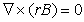(1)

where H, B and r are respectively magnetic field intensity vector, magnetic flux density vector and reluctivity tensor. Common model of the reluctivity tensor is :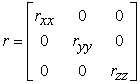(2)

where rxx, ryy and rzz are non-linear function of B. Z axis is considered perpendicular to the surface of laminations of the core. rxx and ryy are assumed independent of z direction.

Since the transformer core is made of many high permeable sheets (maximum thickness 0.3 mm), separated from each other by the insulation coating with a thickness of less than 10 μm, the magnetic field can only be considered in sheets . The normal magnetic flux density is negligible and usually less than 4 mT . In this paper, the direction of magnetic flux density assumed to be perpendicular to the core sheets’ surface in the whole core structure, except for the joints, in which it is assumed to be zero. This assumption, significantly, reduces the problem complexity and the original 3-D problem can be substituted by some 2-D analytical from models and the core loss estimation becomes independent the number of pockets in the assembled core. With this assumption, Equation (1) can be rewritten as follows: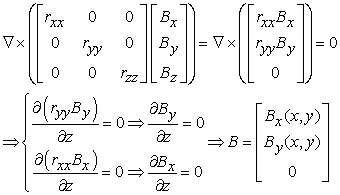(3)

As it can be seen in Equ. (3), Bx(x,y) and By(x,y) are independent of the z direction. Therefore, only the largest pocket of the transformer core is simulated in xy-plane model. The xy-plane loss is calculated by integrating the spatial loss distribution on main pocket over the whole core structure. Therefore, core loss evaluation is independent of the number of the pockets, unlike the conventional methods. Thus, computation speed is increased by a factor of the number of pockets. Figure 2 shows xy-plane model of three phase stacked core.

The imposed voltages on the transformer coils have a sinusoidal form, under the standard test condition. Flux boundary conditions are used to guarantee the sinusoidal waveform for the applied voltage. Using Faraday’s equation, the total flux in the cross section of the limbs is a given value at each instant of time as follows .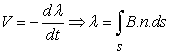(4)

where V,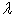, n and s are sinusoidal imposed voltage, total flux, normal vector of limb cross section and area of limb cross section, respectively.

The presence of core stacking holes can increase the core loss up to 3% . The holes distort the flux wave shapes and result in higher harmonics and so higher losses . For example, Figure 3 shows effect of a hole on distortion of flux density across sheet width. The holes are modeled in this simulation for more accurate results unlike .

FEA program solves 2-D model of main pocket and returns the magnetic flux density distribution in the xy-plane at any instant of time. In Figure 4 the magnetic flux density distribution at two different instants of time is shown.

To calculate the magnetic flux density variation with respect to time at any element of transformer core, similar to , 12 equal space instants of time are assumed. The xy-plane model is solved for these 12 instants with their specific flux boundary conditions. For example, Figure 5 shows magnetic flux density waveforms at P point in the rolling direction and the cross rolling direction as indicated in Figure 2.

2.2. Z-Plane Modeling

Due to presence of air gaps at the joints, magnetic flux travels between the overlapping laminations of yokes and limbs and when these laminations are saturated, flux crosses the air gaps . This flux distortion causes additional localized losses. This additional loss cannot be evaluated from xy-plane models. A suitable method is introduced to solve this problem in modeling the sheets in the z-plane in the vicinity of the joints [2, 4, 10, 14]. In Figure 6, the magnetic flux density behavior for Single Step Lap (SSL) and Multi-Step Lap (MSL) structures, using CRGO steel while, operating under a magnetic flux density of 1.7 Tesla is shown.

Figure 6. Magnetic flux distribution at area of joints due to presence of air gaps, a) Single Step Lap, b) Multi-Step Lap

The variation of magnetic flux density in the SSL and MSL joints (with different steps) along the line L is plotted and shown in Figure 7. As it is shown in Figure 7, the flux distortion takes place only in a distance of several centimeters around the air gaps. Therefore the application of z-plane model is limited to this area and it is independent of the overall structure of transformer core.

In MSL joints, the magnetic flux density in the air gaps reduces to about zero in comparison with the SSL joints. The magnitudes of core sheets saturation are decreased; however the area of the saturated region is increased as shown in Figure 7. However, usually MSL joints are known to reduce the loss in the core joints.

For several magnetic flux densities, 2-D structure of Figure 6 is solved and the extra localized joint loss is calculated.

To calculate total z-plane loss, magnetic induction waveforms at the L-joints and T-joints are evaluated from Solved xy-plane models. Then ALJL is integrated along the length of joints as follows.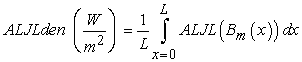(5)

where ALJLden, Bm(x) are the density of additional localized joint loss over the area of joints and maximum magnetic induction at the length of x along joints.

Total z-plane loss can be obtained by multiplying ALJLden by the area of joints cross section.

### 3. Curve Fitting of Reluctivity

In numerical modeling of the non-linear behavior of the transformer core material, often a mathematical relation between relative permeability and the flux density is required. Different fitting methods, such as the hyperbolic equations  are presented in past, in the literature. In this paper, Gaussian curve fitting with 3 terms is used as Equ. (6) .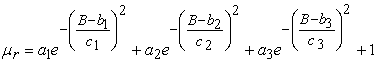(6)

where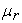is relative permeability. ai, bi and ci are parameters which are determined with curve fitting algorithm. Gaussian method has a powerful ability for modeling the extremely non-linear behavior of material permeability. For example, Figure 8 shows the measured data and the fitted curve for M5 CRGO Steel.

### 4. Core Loss Model for Non-Sinusoidal Waveforms

As shown in Figure 5, the instantaneous magnetic flux density waveforms in the elements of transformer core demonstrate a non-sinusoidal variation. Therefore, in this modeling the equations related to the sinusoidal waveforms cannot be used. In recent years, accurate equations are developed to model the core loss when a non-sinusoidal flux density waveform exists in the magnetic materials. In this paper, to calculate the losses in each element of the transformer core, Equ. (7), as proposed by [15, 16], used to determine the losses: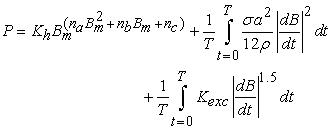(7)

where maximum flux density, the sheet thickness, its conductivity, its mass density and the time period are represented by bm, a, σ, ρ and T respectively. Kh, Kexc and na,b,c are the constant coefficients of the magnetic material. These coefficients can be obtained using the specific loss of the magnetic material in specific frequencies and specific magnetic flux densities.

Using Equ. (7), spatial distribution of core loss of xy-plane is shown in Figure 9. Stacking holes are ignored in Figure 9 for a better visualization.

Figure 9. Spatial distribution of core loss of xy-plane model in upper half of 3-phase 3-limb core

### 5. Verification of Calculations

To verify proposed method, this model is implemented on 12 commercial size three-phase three-limb distribution transformer samples and the results are compared with the factory measurements. For every distribution transformer, several numbers of a specific size are tested and the average core loss is used as the measured loss. The selected samples have various core structures, rated powers, rated voltages and the design magnetic flux density. In Table 1 some samples of the results are shown. The average accuracy of the new method was 0.28% and its standard deviation was 3.41%. Thus, compared to the conventional method, such as , this method increases the speed of calculation by a factor of 8 while the average error increases only by 0.08%.

#### Table 1. Comparison of the Proposed Method Outputs for Various Commercial Size Transformers, Against the MeasurementsDownload asPowerPoint Slide

Veiw figureView current table in a new window

### 6. Summary and Conclusion

Requirement of a calculation tool to accurately determine the impact of design parameters on the core loss optimization seems inevitable for a transformer manufacturer. In this paper an accurate and fast method for core loss estimation is presented. In this method, almost, all of the core design parameters such as; the geometrical parameters, the joints parameters and the stacking holes effect are taken into account. Therefore, the core optimization software on one hand can control all the design parameters, and on the other hand, there is more time available to check various modes of the design parameters. In the later works, the effects of various design parameters on the core loss must be studied.

### Statement of Competing Interests

The authors have no competing interests.

### References

  Daut, I., Ahmad, D.M.M., Zakaria, S., and Taib, S., “Comparison on losses and flux distribution between two 3-phase distribution transformers 1000 KVA assembled with air gap and without air gap of transformer core lamination”, IEEE Asia-Pacific conf. Applied Electromagnetics (APACE 2007), Dec. 4-6, 2007.In article  Ilo, A., Pfutzner, H., Nakata, T., “Critical induction-a key quantity for the optimisation of transformer core operation”, Journal of Magnetism and Magnetic Materials, 215-216. 637-640. 2000.In article CrossRef  Targosz, R., and Topalis, F.V., “Energy efficiency of distribution transformers in Europe”, 9th int. conf. Electrical and Power Quality and Utilization (EPQU 2007), Oct. 9-11, 2007, Barcelona.In article  Olivares, J.C., Liu, Y., Canedo, J., Escarela-Perez, R., Driesen, J., and Moreno, P., “Reducing losses in distribution transformers”, IEEE Transaction on Power Delivery, 18 (3). 821-826. Jul. 2003.In article CrossRef  Amoiralis, E.I., Tsili, M.A., Georgilakis, P.S., Kladas, A.G., and Souflaris, A.T., “A parallel mixed integer programming-finite element method technique for global design optimization of power transformers”, IEEE Transaction on Magnetics, 44 (6). 1022-1025. Jun. 2008.In article CrossRef  Kefalas, T.D., Georgilakis, P.S., Kladas, A.G., Souflaris, A.T., and Paparigas, D.G., “Multiple grade lamination wound core: a novel technique for transformer iron loss minimization using simulated annealing with restarts and an anisotropy model”, IEEE Transaction on Magnetics, 44 (6). 1082-1085. Jun. 2008.In article CrossRef  Hernandez, C., Arjona, M.A., and Dong, S.H., “Object-oriented knowledge-based system for distribution transformer design”, IEEE Transaction on Magnetics, 44 (10). 2332-2337. Oct. 2008.In article CrossRef  teNyenhuis, E.G., Girgis, R., and Mechler, G.F., “Other factors contributing to the core loss performance of power and distribution transformers”, IEEE Transaction on Power Delivery, 16 (4). 648-653. Oct. 2001.In article CrossRef  Valkovic, Z., and Rezic, A., “Improvement of transformer core magnetic properties using the step-lap design”, Journal of Magnetism and Magnetic Materials, 112. 413-415. 1992.In article CrossRef  Mechler, G.F., and Girgis, R.S., “Calculation of spatial loss distribution in stacked power and distribution transformer cores”, IEEE Transaction on Power Delivery, 13 (2). 532-537. Apr. 1998.In article CrossRef  National Electrical Manufacturers Association, “NEMA TP2: Standard Test Method for Measuring the Energy Consumption of Distribution Transformers”, Jan. 2005.In article  Prieto, R., Cobos, J.A., Bataller, V., Garcia, O., and Ucedci, J., “Study of toroidal transformers by means of 2D approaches”, 28th Annu. IEEE Conf. Power Electronics Specialists (PESC 1997), Jun. 22-27, 1997.In article  Valkovic, Z., “Additional losses in three-phase transformer cores”, Journal of Magnetism and Magnetic Materials, 41. 424-426. Feb. 1984.In article CrossRef  Mechler, G.F., and Girgis, R.S., “Magnetic flux distribution in transformer core joints”, IEEE. Transaction on Power Delivery, 15 (1). 198-203. Jan. 2000.In article CrossRef  Mousavi, S.A., “Harmonic core losses calculation in power transformers”, M.Sc. thesis, University of Tehran, Tehran, Iran, 2008.In article  Roshen, W.A., “A practical, accurate and very general core loss model for non-sinusoidal waveforms”, IEEE Transaction on Power Electronics, 22 (1). 30-40. Jan. 2007.In article CrossRef  Moses, A.J., “Prediction of core losses of three phase transformers from estimation of the components contributing to the building factor”, Journal of Magnetism and Magnetic Materials, 254-255. 615-617. 2003.In article CrossRef  Khelil, M., Elleuch, M., “Modeling of the air-gaps of overlapped joints in three-phase transformer iron core for using by FEM”, IEEE Multi-Conference on Systems, Signals and Devices (SSD 2009), Mar. 23-26, 2009.In article  Hajipour, E., Rezaei, P., Vakilian, M., Ghafouri, M., “Power Transformer No-Load Loss Prediction with FEM Modeling and Building Factor Optimization,” Journal of Electromagnetic Analysis and Applications, 3 (10). 430-438. 2011.In article CrossRef